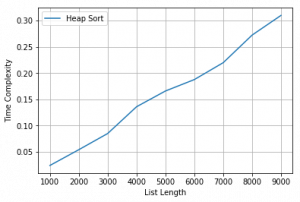# Python Code for time Complexity plot of Heap Sort

Prerequisite : HeapSort
Heap sort is a comparison based sorting technique based on Binary Heap data structure. It is similar to selection sort where we first find the maximum element and place the maximum element at the end. We repeat the same process for remaining element.

We implement Heap Sort here, call it for different sized random lists, measure time taken for different sizes and generate a plot of input size vs time taken.

 `# Python Code for Implementation and running time Algorithm  ` `# Complexity plot of Heap Sort  ` `# by Ashok Kajal  ` `# This python code intends to implement Heap Sort Algorithm ` `# Plots its time Complexity on list of different sizes ` ` `  `# ---------------------Important Note ------------------- ` `# numpy, time and matplotlib.pyplot are required to run this code  ` `import` `time ` `from` `numpy.random ``import` `seed ` `from` `numpy.random ``import` `randint ` `import` `matplotlib.pyplot as plt ` ` `  ` `  `# find left child of node i ` `def` `left(i): ` `    ``return` `2` `*` `i ``+` `1` ` `  `# find right child of node i ` `def` `right(i): ` `    ``return` `2` `*` `i ``+` `2` ` `  `# calculate and return array size ` `def` `heapSize(A): ` `    ``return` `len``(A)``-``1` ` `  ` `  `# This function takes an array and Heapyfies ` `# the at node i ` `def` `MaxHeapify(A, i): ` `    ``# print("in heapy", i) ` `    ``l ``=` `left(i) ` `    ``r ``=` `right(i) ` `     `  `    ``# heapSize = len(A) ` `    ``# print("left", l, "Rightt", r, "Size", heapSize) ` `    ``if` `l<``=` `heapSize(A) ``and` `A[l] > A[i] : ` `        ``largest ``=` `l ` `    ``else``: ` `        ``largest ``=` `i ` `    ``if` `r<``=` `heapSize(A) ``and` `A[r] > A[largest]: ` `        ``largest ``=` `r ` `    ``if` `largest !``=` `i: ` `       ``# print("Largest", largest) ` `        ``A[i], A[largest]``=` `A[largest], A[i] ` `       ``# print("List", A) ` `        ``MaxHeapify(A, largest) ` `     `  `# this function makes a heapified array ` `def` `BuildMaxHeap(A): ` `    ``for` `i ``in` `range``(``int``(heapSize(A)``/``2``)``-``1``, ``-``1``, ``-``1``): ` `        ``MaxHeapify(A, i) ` `         `  `# Sorting is done using heap of array ` `def` `HeapSort(A): ` `    ``BuildMaxHeap(A) ` `    ``B ``=` `list``() ` `    ``heapSize1 ``=` `heapSize(A) ` `    ``for` `i ``in` `range``(heapSize(A), ``0``, ``-``1``): ` `        ``A[``0``], A[i]``=` `A[i], A[``0``]  ` `        ``B.append(A[heapSize1]) ` `        ``A ``=` `A[:``-``1``] ` `        ``heapSize1 ``=` `heapSize1``-``1` `        ``MaxHeapify(A, ``0``) ` `         `  ` `  `# randomly generates list of different ` `# sizes and call HeapSort funtion ` `elements ``=` `list``() ` `times ``=` `list``() ` `for` `i ``in` `range``(``1``, ``10``): ` ` `  `    ``# generate some integers ` `    ``a ``=` `randint(``0``, ``1000` `*` `i, ``1000` `*` `i) ` `    ``# print(i) ` `    ``start ``=` `time.clock() ` `    ``HeapSort(a) ` `    ``end ``=` `time.clock() ` ` `  `    ``# print("Sorted list is ", a) ` `    ``print``(``len``(a), ``"Elements Sorted by HeapSort in "``, end``-``start) ` `    ``elements.append(``len``(a)) ` `    ``times.append(end``-``start) ` ` `  `plt.xlabel(``'List Length'``) ` `plt.ylabel(``'Time Complexity'``) ` `plt.plot(elements, times, label ``=``'Heap Sort'``) ` `plt.grid() ` `plt.legend() ` `plt.show() ` `# This code is contributed by Ashok Kajal `

Output :

```Input : Unsorted Lists of Different sizes are Generated Randomly
Output :
1000 Elements Sorted by HeapSort in  0.023797415087301488
2000 Elements Sorted by HeapSort in  0.053856713614550245
3000 Elements Sorted by HeapSort in  0.08474737185133563
4000 Elements Sorted by HeapSort in  0.13578669978414837
5000 Elements Sorted by HeapSort in  0.1658182863213824
6000 Elements Sorted by HeapSort in  0.1875901601906662
7000 Elements Sorted by HeapSort in  0.21982946862249264
8000 Elements Sorted by HeapSort in  0.2724293921580738
9000 Elements Sorted by HeapSort in  0.30996323029421546

Complexity PLot for Heap Sort is Given  Below```

GeeksforGeeks has prepared a complete interview preparation course with premium videos, theory, practice problems, TA support and many more features. Please refer Placement 100 for details

My Personal Notes arrow_drop_upCheck out this Author's contributed articles.

If you like GeeksforGeeks and would like to contribute, you can also write an article using contribute.geeksforgeeks.org or mail your article to contribute@geeksforgeeks.org. See your article appearing on the GeeksforGeeks main page and help other Geeks.

Please Improve this article if you find anything incorrect by clicking on the "Improve Article" button below.

Improved By : Akanksha_Rai